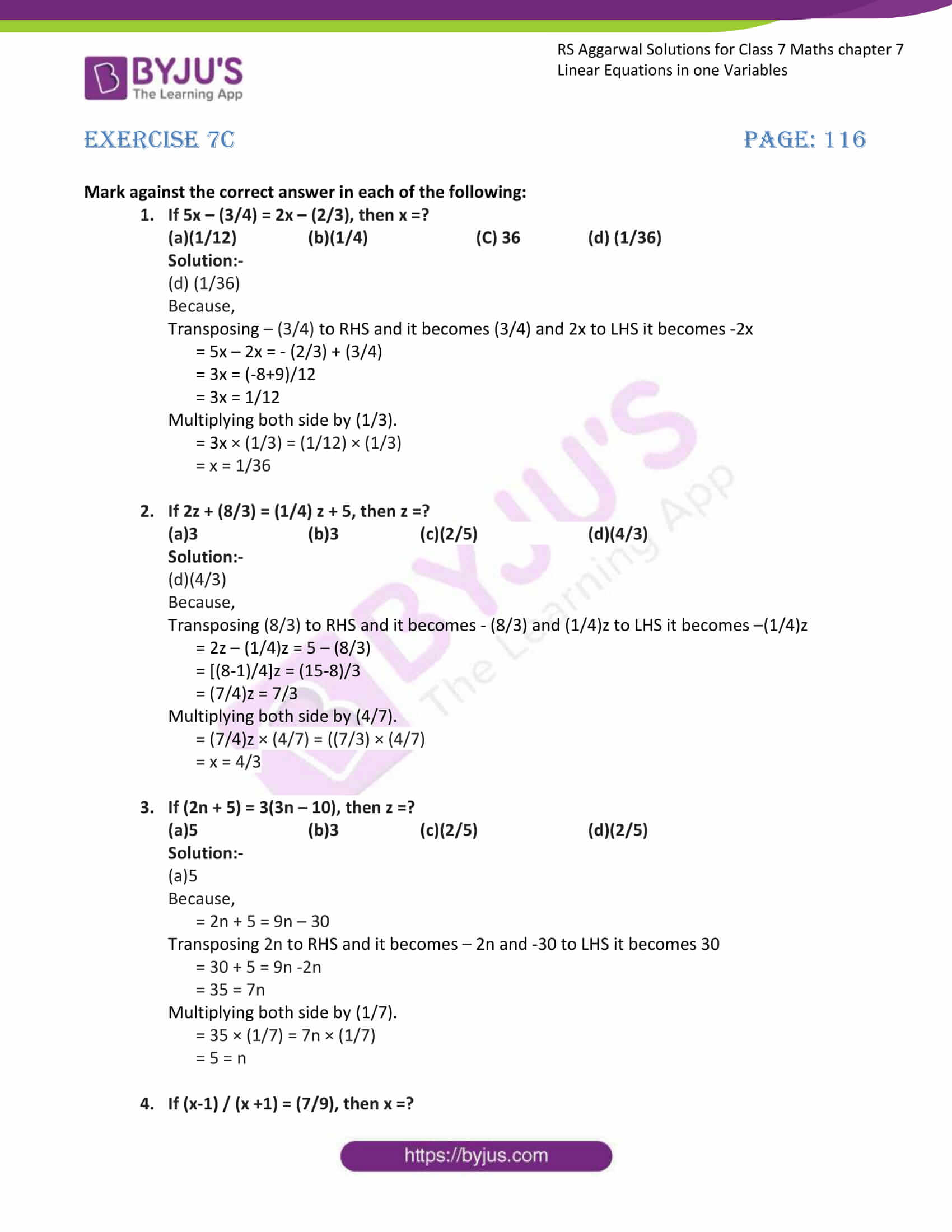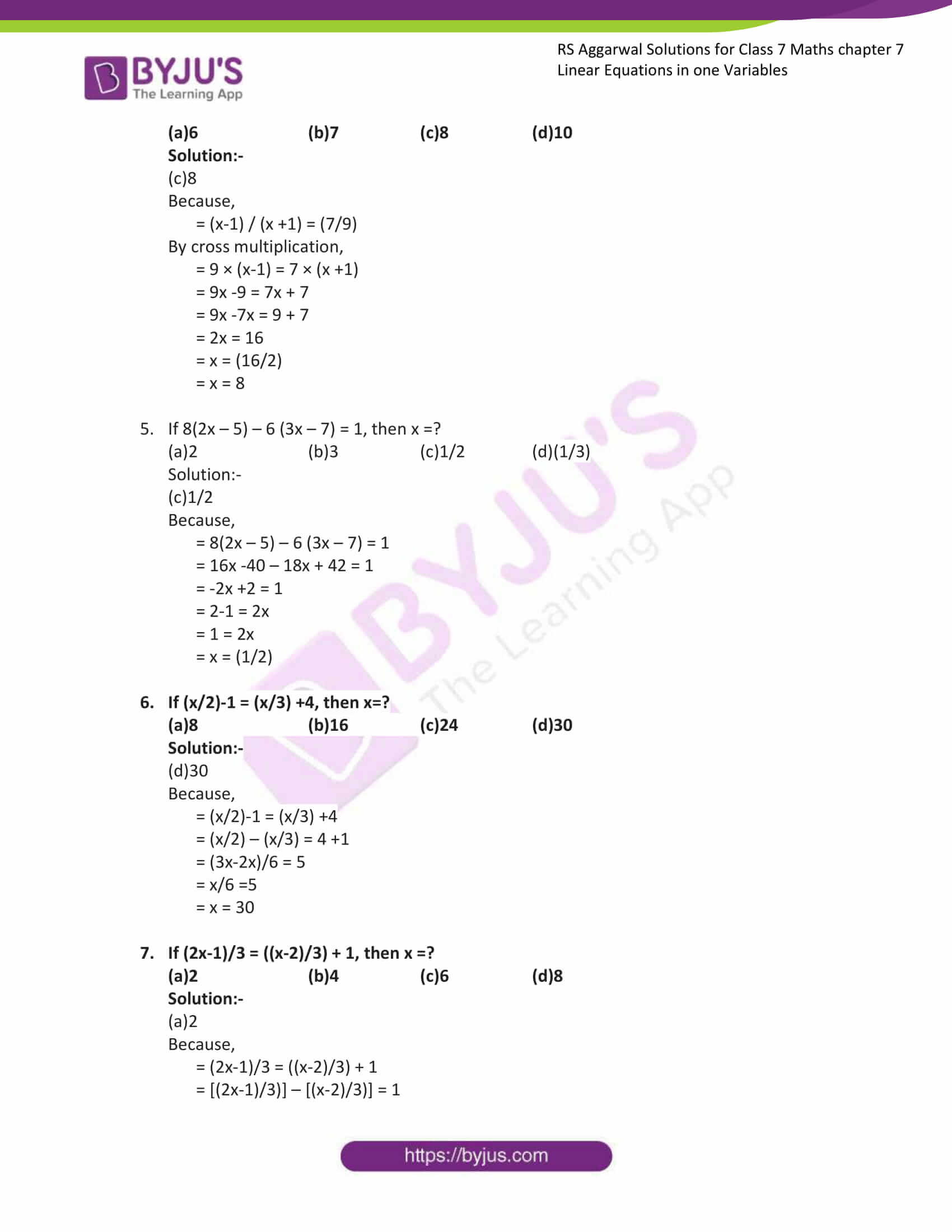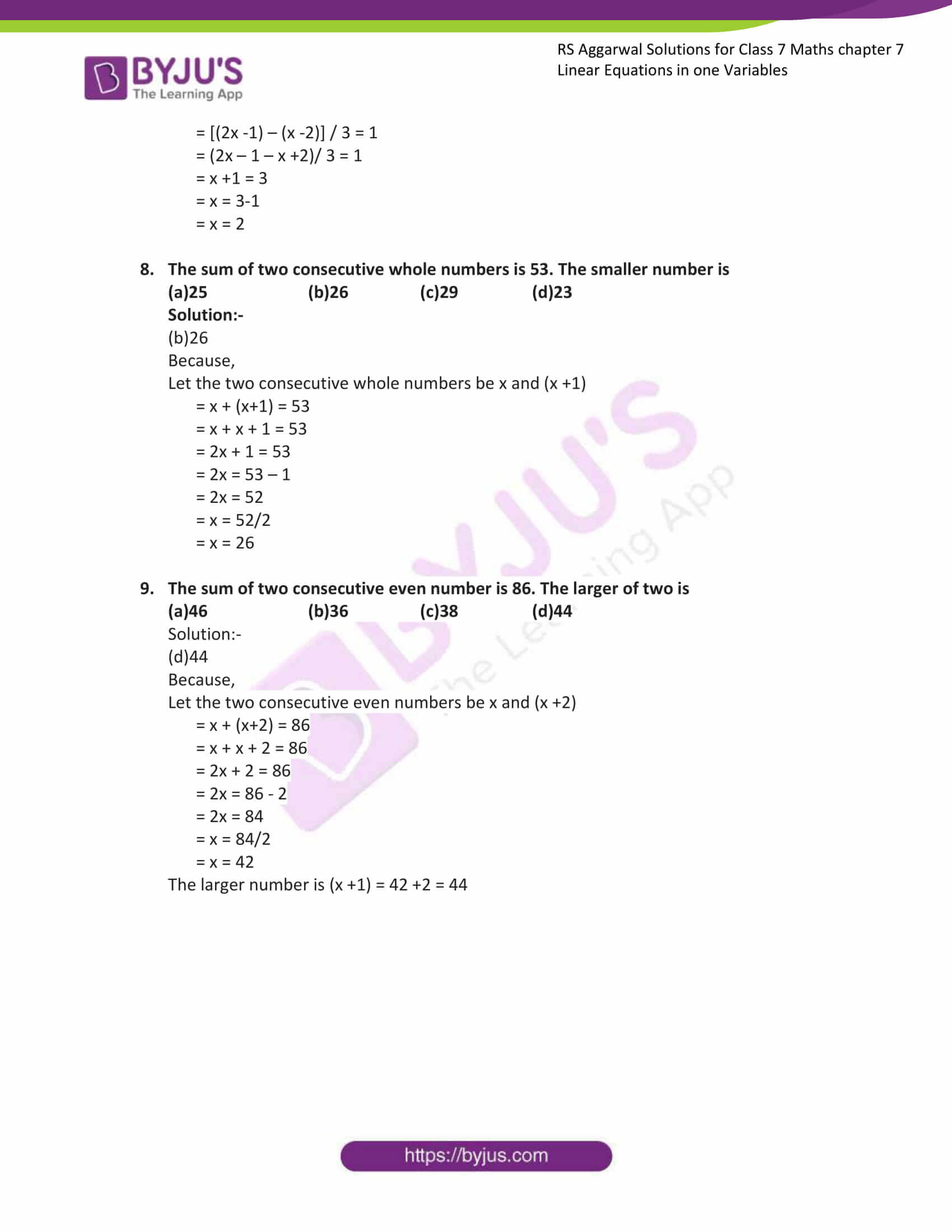# RS Aggarwal Solutions for Class 7 Maths Exercise 7C Chapter 7 Linear Equations in One Variable

RS Aggarwal Solutions for Class 7 Maths Exercise 7C Chapter 7 Linear Equations in One Variable in simple PDF are given here for download. This exercise contains objective type questions. These questions are simple, but students have to think in different ways to get answers. Students of Class 7 are suggested to solve RS Aggarwal Class 7 Solutions Chapter 7 to understand and crack these problems. Our expert tutors have prepared the RS Aggarwal Solutions for Class 7 Maths Chapter 7 Linear Equations in One Variable.

## Download the PDF of RS Aggarwal Solutions For Class 7 Maths Chapter 7 Linear Equations in One Variable – Exercise 7C### Access answers to Maths RS Aggarwal Solutions for Class 7 Chapter 7 – Linear Equations in One Variable Exercise 7C

Mark against the correct answer in each of the following:

1. If 5x – (3/4) = 2x – (2/3), then x =?

(a)(1/12) (b)(1/4) (C) 36 (d) (1/36)

Solution:-

(d) (1/36)

Because,

Transposing – (3/4) to RHS and it becomes (3/4) and 2x to LHS it becomes -2x

= 5x – 2x = – (2/3) + (3/4)

= 3x = (-8+9)/12

= 3x = 1/12

Multiplying both side by (1/3).

= 3x × (1/3) = (1/12) × (1/3)

= x = 1/36

2. If 2z + (8/3) = (1/4) z + 5, then z =?

(a)3 (b)3 (c)(2/5) (d)(4/3)

Solution:-

(d)(4/3)

Because,

Transposing (8/3) to RHS and it becomes – (8/3) and (1/4)z to LHS it becomes –(1/4)z

= 2z – (1/4)z = 5 – (8/3)

= [(8-1)/4]z = (15-8)/3

= (7/4)z = 7/3

Multiplying both side by (4/7).

= (7/4)z × (4/7) = ((7/3) × (4/7)

= x = 4/3

3. If (2n + 5) = 3(3n – 10), then z =?

(a)5 (b)3 (c)(2/5) (d)(2/5)

Solution:-

(a)5

Because,

= 2n + 5 = 9n – 30

Transposing 2n to RHS and it becomes – 2n and -30 to LHS it becomes 30

= 30 + 5 = 9n -2n

= 35 = 7n

Multiplying both side by (1/7).

= 35 × (1/7) = 7n × (1/7)

= 5 = n

4. If (x-1) / (x +1) = (7/9), then x =?

(a)6 (b)7 (c)8 (d)10

Solution:-

(c)8

Because,

= (x-1) / (x +1) = (7/9)

By cross multiplication,

= 9 × (x-1) = 7 × (x +1)

= 9x -9 = 7x + 7

= 9x -7x = 9 + 7

= 2x = 16

= x = (16/2)

= x = 8

5. If 8(2x – 5) – 6 (3x – 7) = 1, then x =?

(a)2 (b)3 (c)1/2 (d)(1/3)

Solution:-

(c)1/2

Because,

= 8(2x – 5) – 6 (3x – 7) = 1

= 16x -40 – 18x + 42 = 1

= -2x +2 = 1

= 2-1 = 2x

= 1 = 2x

= x = (1/2)

6. If (x/2)-1 = (x/3) +4, then x=?

(a)8 (b)16 (c)24 (d)30

Solution:-

(d)30

Because,

= (x/2)-1 = (x/3) +4

= (x/2) – (x/3) = 4 +1

= (3x-2x)/6 = 5

= x/6 =5

= x = 30

7. If (2x-1)/3 = ((x-2)/3) + 1, then x =?

(a)2 (b)4 (c)6 (d)8

Solution:-

(a)2

Because,

= (2x-1)/3 = ((x-2)/3) + 1

= [(2x-1)/3)] – [(x-2)/3)] = 1

= [(2x -1) – (x -2)] / 3 = 1

= (2x – 1 – x +2)/ 3 = 1

= x +1 = 3

= x = 3-1

= x = 2

8. The sum of two consecutive whole numbers is 53. The smaller number is

(a)25 (b)26 (c)29 (d)23

Solution:-

(b)26

Because,

Let the two consecutive whole numbers be x and (x +1)

= x + (x+1) = 53

= x + x + 1 = 53

= 2x + 1 = 53

= 2x = 53 – 1

= 2x = 52

= x = 52/2

= x = 26

9. The sum of two consecutive even number is 86. The larger of two is

(a)46 (b)36 (c)38 (d)44

Solution:-

(d)44

Because,

Let the two consecutive even numbers be x and (x +2)

= x + (x+2) = 86

= x + x + 2 = 86

= 2x + 2 = 86

= 2x = 86 – 2

= 2x = 84

= x = 84/2

= x = 42

The larger number is (x +1) = 42 +2 = 44

### Access other exercises of RS Aggarwal Solutions For Class 7 Chapter 7 – Linear Equations in One Variable

Exercise 7B Solutions